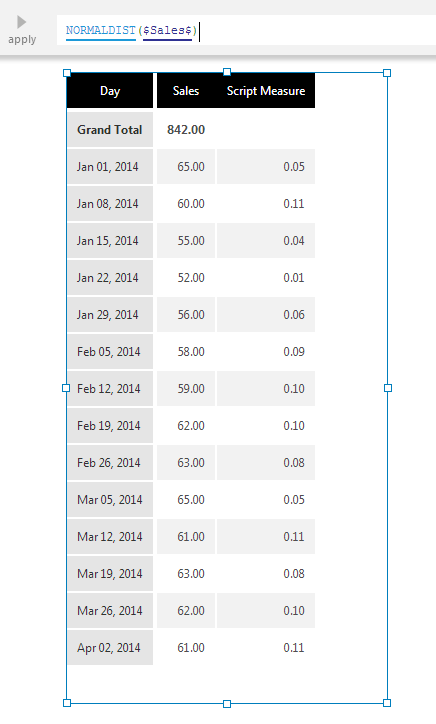# Normal Distribution

Contents[Hide]

The Normal Distribution function calculates the standard normal distribution for an input data series.

Specifically, the function computes the relative likelihood that a continuous random variable would equal the input value if the series had a symmetrical "bell-curved" distribution.Normal Distribution output shown on the Script Measure column

## 1. Syntax

```NORMALDIST(d0,Alignments...)
```

## 2. Input

The Normal Distribution function requires the following input series:

• d0 - Input data values - The set of data values for which the Normal Distribution is calculated.

## 3. Parameters

The Normal Distribution function requires the following parameters:

• Alignments... (One or More, Optional) – Hierarchy placeholders to be used as the alignment axis.

## 4. Output

The Normal Distribution function generates the following outputs:

• Normal Distribution - The Normal Distribution result set.

## 5. See also

Dundas Data Visualization, Inc.
500-250 Ferrand Drive
Toronto, ON, Canada
M3C 3G8

North America: 1.800.463.1492
International: 1.416.467.5100

Dundas Support Hours: 7am-6pm, ET, Mon-Fri